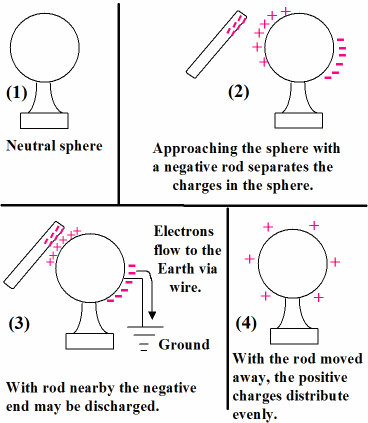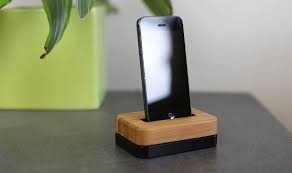×#### Thank you for registering.

One of our academic counsellors will contact you within 1 working day.

Click to Chat

1800-1023-196

+91-120-4616500

CART 0

• 0

MY CART (5)

Use Coupon: CART20 and get 20% off on all online Study Material

ITEM
DETAILS
MRP
DISCOUNT
FINAL PRICE
Total Price: Rs.

There are no items in this cart.
Continue Shopping• Complete Physics Course - Class 11
• OFFERED PRICE: Rs. 2,968
• View Details

```Charging by Induction

Table of Content

What is Induction?

How Charge Carriers move

How can we charge a Single Sphere by Induction Method?

How can we charge Two Spheres by Induction Method?

Why we need Charging by Induction Method

How can we find that a charge is present on an Object?

Real Life Example of Charging by Induction Method

What is the difference between Charging by Induction and Charging by Conduction?

What is the difference between Charging by Induction and Charging by Friction?

In this article, we will discuss how we can charge a body or object through induction method. There are three methods to charge an object:

Charge by Friction

Charge by Conduction

Charge by Induction

We will discuss in detail how we can charge a body through Induction method.

What is Induction?

Induction is the process in which, when we bring two substances near to each other due to the property of induction there is movement of charge carriers from one substance to another substance but the objects are not physically touch each other.

How Charge Carriers move

Let us suppose that sphere have negative charge on former side and positive charge on latter side. We bring negative rod near to former side of sphere then negative charge of sphere repel by negative charge of rod. In this way, there is movement of charge. When we have to charge an object by induction method, then induced charge produce on the object.

How can we charge a Single Sphere by Induction Method?Let us consider a neutral sphere made with the metal. Since metal is a good conductor of electricity, so we have choosen the object made from metal. The neutral sphere is placed on an insulator sheet as shown in diagram (right), so that the charge doesn’t flow out from the system. As we can see in the figure 2; there is equal amount of positive and negative charge on the sphere (number of positive charge = 6 = number of negative charge) and hence, we can say that the object is neutral as shown in the first part of diagram.

In the second part of the diagram, we bring the negatively charged rod (rod shown in figure 3 & 4 having negative charge) near the sphere. Sphere have both positive and negative charge and as the rod is brought near to the sphere, the negative charge in sphere are repelled by the rod (due to same charge) and move to the outer side of sphere. Number of positive charges are equal to the number of negative charges. We can say, that total charge on a system is zero.

In the third part of diagram, we ground the sphere and negative charge move to the ground.  So only positive charge remains left. In the fourth part of diagram, positive charge evenly spread on the sphere.

Note: In Charge by Induction method objects are not in physically contact with each other while in remaining two methods objects are in physically contact with each other.

How can we charge Two Spheres by Induction Method?

Let us consider two spheres A and B they are placed on an insulating stand so that net charge remains in the system. Both the spheres are in contact with each other as shown in diagram (i).If we place a positive rod near the sphere A then positive charge from sphere A are repelled by rod and move to the sphere B and negative charge from sphere B move to the sphere A as shown in diagram (ii).

If we separate both the spheres then in sphere A only negative charge is present and in sphere B only positive charge is present and net amount of charge in both the sphere are equal as shown in diagram (iii).

In the diagram (iv) there is equally uniform distribution of charges.

Why we need Charging by Induction Method

It is only method in which we can charge a object without touch to a body.

How can we find that a charge is present on an Object?

We use Electroscope to measure the charge on an object. is a device to find the presence of charge in an object.

Real Life Example of Charging by Induction Method:

In commercial products, the induction charging process is governed with the help of induction coils. In smartphones, both the phone and the charging dock contain induction coils of iron wrapped with copper wire. When we place the phone on the charging dock an electromagnetic field is produced between the induction coils. Once the electromagnetic field is produced, electricity is able to pass between the two induction coils, charging the phone wirelessly.

What is the difference between Charging by Induction and Charging by Conduction?

In Charging by Induction method objects do not come in contact with each other, while in Charging by Conduction method object physically come in contact with each other.

What is the difference between Charging by Induction and Charging by Friction?

In Charging by Friction method, charge is produced on a body by rubbing the other object while in Charging by Induction method charge is produced on a body by bringing the another body near to that object.

Q1. What does it mean to induce a charge?

Sol. Induce a charge means produce a charge of opposite sign but equal magnitude on an object without physically touch the objects.

Q2. What is meant by electrostatic induction?

Sol. Electrostatic induction is the method to create electricity in a material by bringing the electrically charged body near to that material.Q3. What is electric induction?

Sol. Electric induction is the method that is used to explain that how electric generators like microphone, smartphones charge with the help of induction method. When we placed a smartphone on dock then electromagnetic field is produced between two objects (dock and phone) due to induction charge from dock transfer to the phone and phone get charged.

Q4. How do you charge an electroscope by induction?

Sol. Electroscope is an instrument used to detect the charge produce by induction method.  From the induction process we charge an object and electroscope tell us about the nature of charge.

Q5. What is the basic difference between charging by induction and charging by friction method?

Sol. Charging by friction method we rub the two bodies and after rubbing the charge on one body is transferred to the charge on another body while charging by induction method is transfer of charge from one object to another when two bodies are not in physically contact each other.

Q6. Which instrument is used to detect the nature of charge?

Sol. Electroscope is an instrument used to detect nature of charge.

Q7. Suppose we want that negative charge spread on sphere then which rod we will bring in contact with sphere i.e. positive or negative? And why?

Sol. If we want that negative charge is spread on the sphere then we will bring positive rod near to sphere. Because positive charge that is already present on the sphere are repelled by the positive charge of rod and move to the outer side of sphere and if we ground the sphere positive charge move to ground and negative charge is left on the sphere.

Q8. What is the basic difference between charging by induction and charges by conduction method?

Sol. Charging by conduction method is the transfer of charge from one object to another when two objects are in contact with each other while charging by induction method is transfer of charge from one object to another when two bodies are not in physically contact each other.

Q9. How charging by induction method is used in real life?

Sol. Charging by induction method is used in real life in charging microphones, smartphones etc. As induction method is the transfer of charge from one object to another so by placing the phone on dock charge transfer from dock to phone.

Watch this Video for more reference

```### Course Features

• 101 Video Lectures
• Revision Notes
• Previous Year Papers
• Mind Map
• Study Planner
• NCERT Solutions
• Discussion Forum
• Test paper with Video Solution